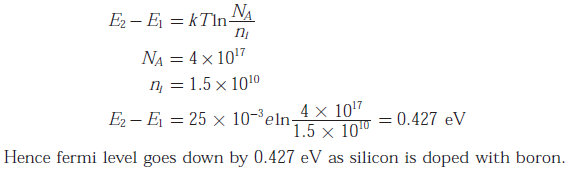# Electronic Devices - Online Test

Q1. Which of the following is true?
Explaination / Solution:

Trivalent impurities are used for making p - type semiconductors. So, Silicon wafer heavily doped with boron is a p+ substrate.

Q2. In the following limiter circuit, an input voltage Vi = 10 sin100πt is applied. Assume that the diode drop is 0.7 V when it is forward biased. When it is forward biased. The zener breakdown voltage is 6.8 V
The maximum and minimum values of the output voltage respectively are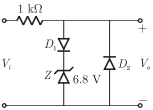Explaination / Solution:
No Explaination.

Q3. A silicon wafer has 100 nm of oxide on it and is furnace at a temperature above 10000 C for further oxidation in dry oxygen. The oxidation rate
Explaination / Solution:
No Explaination.

Q4. The drain current of MOSFET in saturation is given by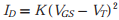where K is a constant.
The magnitude of the transconductance gm is
Explaination / Solution:Q5.
For the circuit shown in the following figure, transistor M1 and M2 are identical NMOS transistors. Assume the M2 is in saturation and the output is unloaded.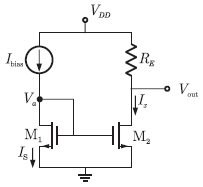The current Ix is related to Ibias as

Explaination / Solution:Q6.
The measured trans conductance gm of an NMOS transistor operating in the linear region is plotted against the gate voltage VG at a constant drain voltage VD. Which of the following figures represents the expected dependence of gm on VG ?
Explaination / Solution:
No Explaination.

Q7. Consider the following circuit using an ideal OPAMP. The I-V characteristic of the diode is described by the relationwhere VT = 25 mV, I0 = 1μ A and V is the voltage across the diode (taken as positive for forward bias). For an input voltage Vi = -1 V, the output voltage V0 is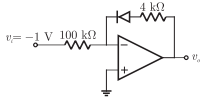Explaination / Solution:Q8.
The OPAMP circuit shown above represents a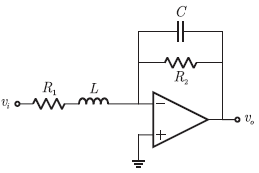Explaination / Solution:and from this equation it may be easily seen that this is the standard form of T.F. of low pass filterand form this equation it may be easily seen that this is the standard form of T.F. of low pass filterQ9. Two identical NMOS transistors M1 and M2 are connected as shown below. Vbias is chosen so that both transistors are in saturation. The equivalent gm of the pair is defied to be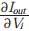at constant Vout
The equivalent gm of the pair isExplaination / Solution:
No Explaination.

Q10. Silicon is doped with boron to a concentration of 4 × 1017 atoms cm3. Assume the intrinsic carrier concentration of silicon to be 1.5 × 1010/cmand the value of kT/q to be 25 mV at 300 K. Compared to undopped silicon, the fermi level of doped silicon
Explaination / Solution: